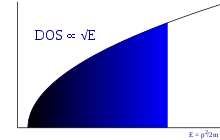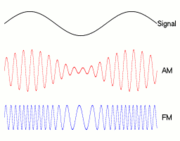# Free electron model

﻿
Free electron modelIn three dimensions, the density of states of a gas of fermions is proportional to the square root of the kinetic energy of the particles.

In solid-state physics, the free electron model is a simple model for the behaviour of valence electrons in a crystal structure of a metallic solid. It was developed principally by Arnold Sommerfeld who combined the classical Drude model with quantum mechanical Fermi-Dirac statistics and hence it is also known as the Drude–Sommerfeld model. The free electron Empty Lattice Approximation forms the basis of the band structure model known as nearly-free electron model. Given its simplicity, it is surprisingly successful in explaining many experimental phenomena, especially

## Ideas and assumptions

As in the Drude model, valence electrons are assumed to be completely detached from their ions (forming an electron gas). As in an ideal gas, electron-electron interactions are completely neglected. The electrostatic fields in metals are weak because of the screening effect.

The crystal lattice is not explicitly taken into account. A quantum-mechanical justification is given by Bloch's Theorem: an unbound electron moves in a periodic potential as a free electron in vacuum, except for the electron mass m becoming an effective mass m* which may deviate considerably from m (one can even use negative effective mass to describe conduction by electron holes). Effective masses can be derived from band structure computations. While the static lattice does not hinder the motion of the electrons, they can well be scattered by impurities and by phonons; these two interactions determine electrical and thermal conductivity (superconductivity requires a more refined theory than the free electron model).

According to the Pauli exclusion principle, each phase space element (Δk)3(Δx)3 can be occupied only by two electrons (one per spin quantum number). This restriction of available electron states is taken into account by Fermi-Dirac statistics (see also Fermi gas). Main predictions of the free-electron model are derived by the Sommerfeld expansion of the Fermi-Dirac occupancy for energies around the Fermi level.

## Energy and wave function of a free electronPlane wave traveling in the x-direction

For a free particle the potential is$V(\bold{r}) = 0$. The Schrödinger equation for such a particle, like the free electron, is$-\frac{\hbar^2}{2m}\nabla^2 \Psi(\bold{r},t) = i\hbar\frac{\partial}{\partial t} \Psi(\bold{r},t)$

The wave function$\Psi(\bold{r},t)$ can be split into a solution of a time dependent and a solution of a time independent equation. The solution of the time dependent equation is$\Psi(\bold{r},t) = \psi(\bold{r}) e^{-i \omega t}$

with energy$E = \hbar \omega$

The solution of the time independent equation is$\psi_{\bold{k}}(\bold{r}) = \frac{1}{\sqrt{\Omega_r}} e^{i\bold{k}\cdot\bold{r}}$

with a wave vector$\bold{k}$. Ωr is the volume of space where the electron can be found. The electron has a kinetic energy$E = \frac{\hbar^2 k^2}{2m}$

The plane wave solution of this Schrödinger equation is$\Psi(\bold{r},t) = \frac{1}{\sqrt{\Omega_r}} e^{i\bold{k}\cdot\bold{r} - i \omega t}$

For solid state and condensed matter physics the time independent solution$\psi_{\bold{k}}(\bold{r})$ is of major interest. It is the basis of electronic band structure models that are widely used in solid-state physics for model Hamiltonians like the nearly-free electron model and the Tight binding model and different models that use a Muffin-tin approximation. The eigenfunctions of these Hamiltonians are Bloch waves which are modulated plane waves.

## Dielectric function of the electron gas

On a scale much larger than the inter atomic distance a solid can be viewed as an aggregate of a negatively charged plasma of the free electron gas and a positively charged background of atomic cores. The background is the rather stiff and massive background of atomic nuclei and core electrons which we will consider to be infinitely massive and fixed in space. The negatively charged plasma is formed by the valence electrons of the free electron model that are uniformly distributed over the interior of the solid. If an oscillating electric field is applied to the solid, the negatively charged plasma tends to move a distance x apart from the positively charged background. As a result the sample is polarized and there will be an excess charge at the opposite surfaces of the sample. The surface charge density is

ρs = − nex

which produces a restoring electric field in the sample$E = \frac{n e x}{\epsilon_0}$

The dielectric function of the sample is expressed as$\epsilon(\omega) = \frac {D(\omega)}{\epsilon_0 E(\omega)} = 1 + \frac {P(\omega)}{\epsilon_0 E(\omega)}$

where D(ω) is the electric displacement and P(ω) is the polarization density.

The electric field and polarization densities are$E(\omega) = E_0 e^{-i \omega t},\quad P(\omega) = P_0 e^{-i \omega t}$

and the polarization per atom with n electrons is

P = − nex

The force F of the oscillating electric field causes the electrons with charge e and mass m to accelerate with an acceleration a$F = e E = m a = m \frac{d^2x}{dt^2}$

which, after substitution of E, P and x, yields an harmonic oscillator equation.

After a little algebra the relation between polarization density and electric field can be expressed as$P(\omega) = - \frac{ne^2}{m\omega^2} E(\omega)$

The frequency dependent dielectric function of the solid is$\epsilon(\omega) = 1 - \frac {n e^2}{\epsilon_0m \omega^2}$

At a resonance frequency ωp, called the plasma frequency, the dielectric function changes sign from negative to positive and real part of the dielectric function drops to zero.$\omega_p = \sqrt{\frac{n e^2}{\epsilon_0 m}}$

This is a plasma oscillation resonance or plasmon. The plasma frequency is a direct measure of the square root of the density of valence electrons in a solid. Observed values are in reasonable agreement with this theoretical prediction for a large number of materials. Below the plasma frequency, the dielectric function is negative and the field cannot penetrate the sample. Light with angular frequency below the plasma frequency will be totally reflected. Above the plasma frequency the light waves can penetrate the sample.

## Solution of the Schrödinger equation

### The Schrödinger equation

For a free particle the potential is$V(\bold{r}) = 0$, so the Schrödinger equation for the free electron is$-\frac{\hbar^2}{2m}\nabla^2 \Psi(\bold{r},t) = i\hbar\frac{\partial}{\partial t} \Psi(\bold{r},t)$

This is a type of wave equation that has numerous kinds of solutions. One way of solving the equation is splitting it in a time-dependent oscillator equation and a space-dependent wave equation like$i\hbar\frac{\partial}{\partial t} \Psi(\bold{r},t) = E \Psi(\bold{r},t)$

and$-\frac{\hbar^2}{2m}\nabla^2 \Psi(\bold{r},t) = E \Psi(\bold{r},t)$

and substituting a product of solutions like$\Psi(\bold{r},t) = \psi(\bold{r}) e^{\alpha t}$

The Schrödinger equation can be split in a time dependent part and a time independent part.

### Solution of the time dependent equation

The peculiar time dependent part of the Schrödinger equation is, unlike the Klein-Gordon equation for pions and most of the other well known wave equations, a first order in time differential equation with a 90o out of phase driving mechanism, while most oscillator equations are second order in time differential equations with 180o out of phase driving mechanisms.

The equation that has to be solved is$i\hbar\frac{\partial}{\partial t} e^{\alpha t} = E e^{\alpha t}$.

The complex (imaginary) exponent is proportional to the energy$\alpha = -\frac{i E}{\hbar}$

The imaginary exponent can be transformed to an angular frequency$E = i \hbar \alpha = \hbar \omega$

The wave function now has a stationary and an oscillating part$\Psi(\bold{r},t) = \psi(\bold{r}) e^{-i \omega t}$

The stationary part is of major importance to the physical properties of the electronic structure of matter.

### Solution of the time independent equation

The wave function of free electrons is in general described as the solution of the time independent Schrödinger equation for free electrons$-\frac{\hbar^2}{2m}\nabla^2 \psi(\bold{r}) = E \psi(\bold{r})$$\nabla^2 = \frac{\partial^2}{{\partial x}^2} + \frac{\partial^2}{{\partial y}^2} + \frac{\partial^2}{{\partial z}^2}$

The wave function can be factorized for the three Cartesian directions$\psi(\bold{r}) = \phi_x(x)\phi_y(y)\phi_z(z)$

Now the time independent Schrödinger equation can be split in three independent parts for the three different Cartesian directions$-\frac{\hbar^2}{2m} \frac{\partial^2}{{\partial x}^2} \phi_x(x) = E_x \phi_x(x)$

As a solution an exponential function is substituted in the time independent Schrödinger equation

ϕx(x) = Nxeκx

The solution of$\frac{\partial^2}{{\partial x}^2} \phi_x(x) = \kappa^2 N_x e^{\kappa x} = -\frac{2m}{\hbar^2} E_x N_x e^{\kappa x}$

gives the exponent$\kappa = i k_x = i \sqrt{\frac{2m E_x}{\hbar^2}}$

which yields the wave equation$\psi(\bold{r}) = N_x N_y N_z e^{i(k_x x + k_y y + k_z z)}$

and the energy$E = \frac{\hbar^2}{2m}(k_x^2 + k_y^2 + k_z^2)$

With the normalization$\int_{\Omega_r} \psi_{\bold{k}}^*(\bold{r})\psi_{\bold{k}'}(\bold{r}) d\bold{r} = \delta_{\bold{k},\bold{k}'}$

and the wave vector length$k = \sqrt{k_x^2 + k_y^2 + k_z^2}$

we arrive at the plane wave solution with a wave function$\psi_{\bold{k}}(\bold{r}) = \frac{1}{\sqrt{\Omega_r}} e^{i\bold{k}\cdot\bold{r}}$

for free electrons with a wave vector$\bold{k}$ and a kinetic energy$E = \frac{\hbar^2 k^2}{2m}$

in which Ωr is the volume of space occupied by the electron.Traveling plane waves restlessly heading for their Final Destination

### The traveling plane wave solution

The product of the time independent stationary wave solution and time dependent oscillator solution$\Psi(\bold{r},t) = \psi(\bold{r}) e^{-i \omega t}$

gives the traveling plane wave solution$\Psi(\bold{r},t) = \frac{1}{\sqrt{\Omega_r}} e^{i\bold{k}\cdot\bold{r} - i \omega t}$

which is the final solution for the free electron wave function.

Wikimedia Foundation. 2010.

### Look at other dictionaries:

• free-electron model — laisvųjų elektronų modelis statusas T sritis fizika atitikmenys: angl. free electron model vok. Modell der freien Elektronen, n rus. модель свободных электронов, f pranc. modèle des électrons libres, m …   Fizikos terminų žodynas

• free-electron model of metals — ▪ physics       in solid state physics, representation of a metallic solid as a container filled with a gas composed of free electrons (i.e., those responsible for high electrical and thermal conductivity). The free electrons, considered… …   Universalium

• Nearly-free electron model — Electronic structure methods Tight binding Nearly free electron model Hartree–Fock method Modern valence bond Generalized valence bond Møller–Plesset perturbat …   Wikipedia

• Model solid approximation — Electronic structure methods Tight binding Nearly free electron model Hartree–Fock method Modern valence bond Generalized valence bond Møller–Plesset perturbation theory …   Wikipedia

• Electron — For other uses, see Electron (disambiguation). Electron Experiments with a Crookes tube first demonstrated the particle nature of electrons. In this illustration, the profile of the cross shaped target is projected against the tube face at right… …   Wikipedia

• Electron liquid — The Electron Liquid is a model system used by physicists to theoretically study interactions among electrons. The uniform electron liquid is a well known model for simple metals like sodium or Aluminum. The ionic charges are assumed to be smudged …   Wikipedia

• Électron — Traduction à relire Electron → …   Wikipédia en Français

• Electron hole — An electron hole is the conceptual and mathematical opposite of an electron, useful in the study of physics and chemistry. The concept describes the lack of an electron. It is different from the positron, which is the antimatter duplicate of the… …   Wikipedia

• Electron configuration — Electron atomic and molecular orbitals Simp …   Wikipedia

• Electron cloud — is a term used, if not originally coined, by the Nobel Prize laureate and acclaimed educator Richard Feynman in The Feynman Lectures on Physics (Feynman2006 Vol 1 lect 6 pg 11) for discussing exactly what is an electron? . This intuitive model… …   Wikipedia